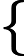Study of mathematics online.
Study math with us and make sure that "Mathematics is easy!"

# Linearly dependent and independent rows

Definition.
A linear combination of rows s1, s2, ..., sl of matrix A is the following expression

α1s1 + α2s2 + ... + αlsl

Definition.
A linear combination of rows is called trivial if all coefficients αi are equal zero simultaneously.
Note.
A trivial linear combination of rows is equal to the zero row.
Definition.
A linear combination of rows is called non-trivial if at least one of the coefficients αi is not equal zero.
Definition.
The system of rows is called linearly dependent, if there is a non-trivial linear combination of rows, which is equal to the zero row.
Definition.
The system of rows is called linearly independent, if only trivial linear combination of rows are equal to the zero row (there is no non-trivial linear combination of rows equal to the zero row).
Note.
System of rows of square matrix are linearly independent if and only if the determinant of the matrix is ​​not equal to zero.
Note.
System of rows of square matrix are linearly dependent if and only if the determinant of the matrix is equals to zero.
Example 1.
Show that the system of rows {s1 = {2   5}; s2 = {4   10}} is linearly dependent.

Solution. Form a linear combination of these rows

α1{2   5} + α2{4   10}

Let us find for what values of α1, α2 the linear combination is equal to the zero row

α1{2   5} + α2{4   10} = {0   0}

This equation is equivalent to the following system of equations:2α1 + 4α2 = 0 5α1 + 10α2 = 0

Divide the first equation by 2, and the second equation by 5:α1 + 2α2 = 0 α1 + 2α2 = 0

The solution of this system may be any number α1 and α2 such that: α1 = -2α2, for example, α2 = 1, α1 = -2, and this means that the rows s1 and s2 are linearly dependent.

Example 2.
Show that the system of lines {s1 = {2   5   1}; s2 = {4   10   0}} is linearly independent.

Solution. Form a linear combination of these rows

α1{2   5   1} + α2{4   10   0}

Let us find for what values of α1, α2 the linear combination is equal to the zero row

α1{2   5   0} + α2{4   10   0} = {0   0   0}

This equation is equivalent to the following system of equations:2α1 + 4α2 = 0 5α1 + 10α2 = 0 α1 + 0α2 = 0

From the third equation gives α1 = 0. Substituting this value in the first and second equation:2·0 + 4α2 = 0 =>4α2 = 0 =>α2 = 0 5·0 + 10α2 = 0 10α2 = 0 α2 = 0 α1 = 0 α1 = 0 α1 = 0

So as a linear combination of rows is equal to zero only when α1 = 0 and α2 = 0, the rows are linearly independent.